# Aim

In this section we want to introduce the concept of adaptive collective variables. These are special variables that are knowledge-based in that are built from a pre-existing notion of the mechanism of the transition under study.

# What happens when in a complex reaction?

When you deal with a complex conformational transition that you want to analyze (or bias), very often you cannot just describe it with a single order parameter.

As an example in Figure belfast-2-cdk-fig I consider a large conformational transition like those involved in activating the kinase via open-close transition of the activation loop. In sticks you see the part involved in the large conformational change, the rest is either keeping the structure and just moving a bit or is a badly resolved region in the X-ray structure. This is a complex transition and it is hard to tell which is the order parameter that best describes the transition.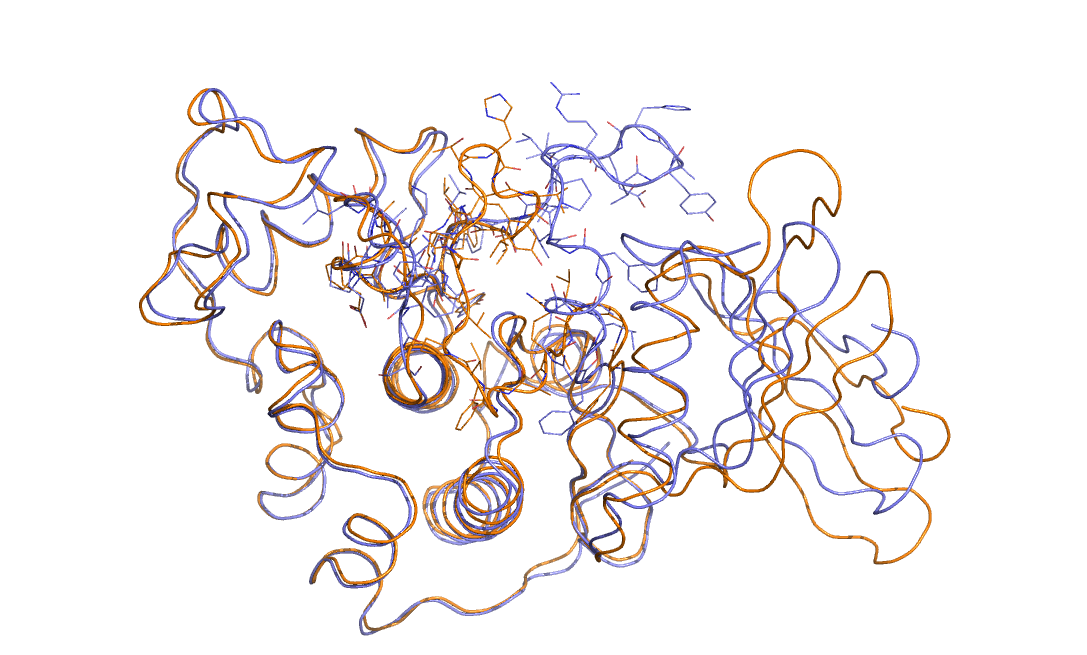CDK2 conformational change, PDB code 2C5X and 2C5Y. The upper loop is radically different. Which torsion or distance is important?

One could identify a distance that can distinguish open from close but

• the plasticity of the loop is such that the same distance can correspond to an almost closed loop and almost open loop. One would like to completely divide these two situations with something which is discriminating what intuitively one would think as open and closed
• the transition state is an important point where one would like to see a certain crucial interaction forming/breaking so to better explain what is really happening. If you capture then hypothetically you would be able to say what is dictating the rate of this conformational transition. A generic distance is a very hybrid measure that is unlikely to capture a salt-bridge formation and a concerted change of many dihedral angles or the change in solvation state that are happening while the transition is happening. All these things are potentially important in such transition but none of them is explaining the whole thing.
So basically in these cases you have to deal with an intrinsic multidimensional collective variable where you would need many dimensions. How would you visualize a 10 dimensional CV where you use many distances, coordination numbers and dihedral angles (ouch, they're periodic too!) ?

Another typical case is the docking of a small molecule in a protein cleft or gorge, which is the mechanism of drug action. This involves specific conformational transition from both the small molecule and the protein as the small molecule approaches the protein cavity. This also might imply a specific change in the behavior when the solvation state changes.
Other typical examples are chemical reactions. Nucleophillic attacks typically happen with a role from the solvent (see some nice paper from Nobel-prize winner Arieh Warshel) and sizable geometric distortions of the neighboring groups.

# Path collective variables

One possibility to describe many different things that happen in a single reaction is to use a dimensional reduction technique and in plumed the simplest example that may show its usefulness can be considered that of the path collective variables.

In a nutshell, your reaction might be very complex and happening in many degree of freedom but intuitively is a sort of track along which the reaction proceeds. So what we need is a coordinate that, given a conformation, just tells which point along the "reactive track" is closest.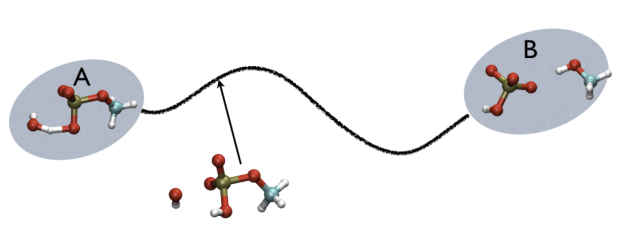Given the reactant and the product, tell if another state is closer to any of the two with a continuous parameter

For example, in Fig. belfast-2-ab-fig, you see a typical chemical reaction (hydrolysis of methyl phosphate) with the two end-points denoted by A and B. If you are given a third point, just by looking at it, you might find that this resembles the reactant more than the product, so, hypothetically, if you would intuitively give a parameter that would be 1 for a configuration in the A state and 2 for a configuration in the B state, you probably would give it something like 1.3, right?

Path collective variables are the extension to this concept in the case you have many conformation that describe your path, and therefore, instead of an index that goes from 1 to 2 you have an index that goes from 1 to $$N$$ , where $$N$$ is the number of conformation that you use in input to describe your path.

From a mathematical point of view, that's rather simple. The progress along the path is calculated with the following equation:

$S(X)=\frac{\sum_{i=1}^{N} i\ \exp^{-\lambda \vert X-X_i \vert }}{ \sum_{i=1}^{N} \exp^{-\lambda \vert X-X_i \vert } }$

where in belfast-2-s-eq the $$\vert X-X_i\vert$$ represents a distance between one configuration $$X$$ which is analyzed and another from the set that compose the path $$X_i$$. The parameter $$\lambda$$ is a positive value that is tuned in a way explained later. here are a number of things to note to make you think that this is exactly what you want.

• The negative exponential function is something that is 1 whenever the value at the exponent is zero, and is progressively smaller when the value is larger than zero (trivially, the case with the value at the exponent larger than zero never occurs since lambda is a positive quantity and the distance is by definition positive).
• Whenever you sit exactly on a specific images $$X_j$$ then all the other terms in the sum disappear (if $$\lambda$$ is large enough) and only the value $$j$$ survives returning exactely $$S(X)=j$$.

In order to provide a value which is continuous, the parameter $$\lambda$$ should be correctly tuned. As a rule of thumb I use the following formula

$\lambda=\frac{2.3 (N-1) }{\sum_{i=1}^{N-1} \vert X_i-X_{i+1} \vert }$

which imply that one should calculate the average distance between consecutive frames composing the path. Note also that this distance should be more or less similar between the frames. Generally I tolerate fluctuation of the order of 10/15 percent tops. If you have larger, then it is better to have a smaller value of $$\lambda$$.

It is important to note that in principle one could even have a specific $$\lambda$$ value associated to each frame of the path but this would provide some distortion in the diffusion coefficient which could potentially harm a straightforward interpretation of the free energy landscape.

So, at this point it is better to understand what is meant with "distance" since a distance between two conformations can be calculated in very many ways. The way we refer here is by using mean square deviation after optimal alignment. This means that at each step in which the analysis is performed, a number N of optimal alignments is performed. Namely what is calculated is $$\vert X-X_i\vert=d(X,X_i)^2$$ where $$d(X,X_i)$$ is the RMSD as defined in what you see here RMSD.

Using the MSD instead of RMSD is sometimes more convenient and more stable (you do not have a denominator that goes to zero in the derivatives when biasing.

Anyway this is a matter of choice. Potentially one could equally employ other metrics like a set of coordination numbers (this was done in the past), and then you would avoid the problem of rototranslations (well, which is not a problem since you have it already in plumed) but for some applications that might become appealing. So in path collective variables (and in all the dimensional reduction based collective variables) the problem is converted from the side of choosing the collective variable in choosing the right way to calculate distances, also called "metrics".

The discussion of this issue is well beyond the topic of this tutorial, so we can move forward in how to tell plumed to calculate the progress along the path whenever the MSD after optimal alignment is used as distance.

p1: PATHMSD REFERENCE=all.pdb LAMBDA=50.0
PRINT ARG=p1.sss,p1.zzz STRIDE=100 FILE=colvar FMT=%8.4f


Note that reference contains a set of PDB, appended one after the other, with a END field. Note that there is no need to place all the atoms of the system in the PDB reference file you provide. Just put the atoms that you think might be needed. You can leave out big domains, solvent and ions if you think that is not important for your use.

Additionally, note that the measure units of LAMBDA are in the units of the code. In gromacs they are in nm^2 while NAMD is Angstrom^2. PATHMSD produces two arguments that can be printed or used in other ActionWithArguments. One is the progress along the path of belfast-2-s-eq, the other is the distance from the closest point along the path, which is denoted with the zzz component. This is defined as

$Z(X)=-\frac{1}{\lambda}\log (\sum_{i=1}^{N} \ \exp^{-\lambda \vert X-X_i \vert })$

It is easy to understand that in case of perfect match of $$X=X_i$$ this equation gives back the value of $$\vertX-X_i\vert$$ provided that the lambda is adjusted correctly.

So, the two variables, put together can be visualized as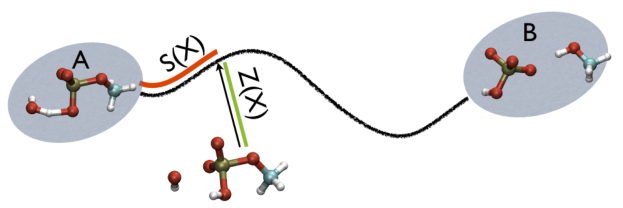The S variable can be thought as the length of the red segment, while the Z variable is the length of the green one.

This variable is important because whenever your simulation is running close to the path (low Z values), then you know that you are reproducing reliably the path you provided in input but if by chance you find some other path that goes, say, from $$S=1, Z=0$$ to $$S=N, Z=0$$ via large Z values, then it might well be that you have just discovered a good alternative pathway. If your path indeed is going from $$S=1, Z=large$$ to $$S=N, Z=large$$ then it might well be that you do not have your reaction accomplished, since your reaction, by definition should go from the reactant which is located at $$S=1, Z=0$$ to the product, which is located at $$S=1, Z=N$$ so you should pay attention. This case is exemplified in Fig. belfast-2-ab-sz-nowhere-fig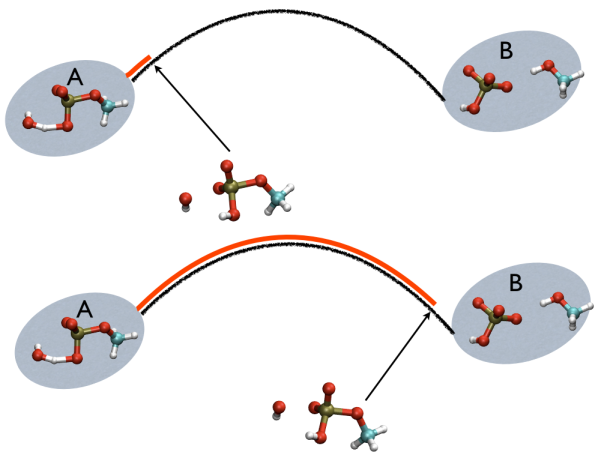The conformation is moving in a small amount and this corresponds to a radical change in S if the distance from the path is large

# A note on the path topology

A truly important point is that if you get a trajectory from some form of accelerated dynamics (e.g. simply by heating) this cannot simply be converted into a path. Since it is likely that your trajectory is going back and forth randomly (not in the case of SMD or US, discussed later), your trajectory might be not topologically suitable. To understand that, suppose you simply collect a reactive trajectory of 100 ps into the reference path you give to the PATHMSD and simply you assign the frame of 1 ps to index 1 (first frame occurring in the reference file provided to PATHMSD), the frame of 2 ps to index 2 and so on : it might be that you have two points which are really similar but one is associated to step, say 5 and the other is associated with frame 12. When you analyze the same trajectory, when you are sitting on any of those points then the calculation of S will be an average like $$S(X)=(5+12)/2=8.5$$ which is an average of the two indexes and is completely misleading since it let you think that you are moving between point 8 and 9, which is not the case. So this evidences that your reference frames should be "topologically consecutive". This means that frame 1 should be the closest to frame 2 and all the other frames should be farther apart. Similarly frame 2 should be equally close (in an RMSD sense) to 1 and 3 while all the others should be farther apart. Same for frame 3: this should be closest to frame 2 and 4 and farther apart from all the others and so on. This is equivalent to calculate an "RMSD matrix" which can be conveniently done in vmd (this is a good exercise for a number of reasons) with RMSD Trajectory tools, by choosing different reference system along the set of reference frames.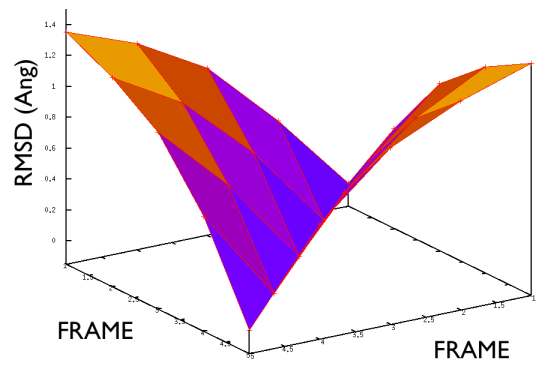A good matrix for path variables has a gull-wing shape, which means that each frame is closest to its neighbor and all the other are far apart

This is shown in Fig. belfast-2-good-matrix-fig where the matrix has a typical gull-wing shape.

On the contrary, whenever you extract the frames from a pdb that you produced via free MD or some biased methods (SMD or Targeted MD for example) then your frame-to-frame distance is rather inhomogeneous and looks something like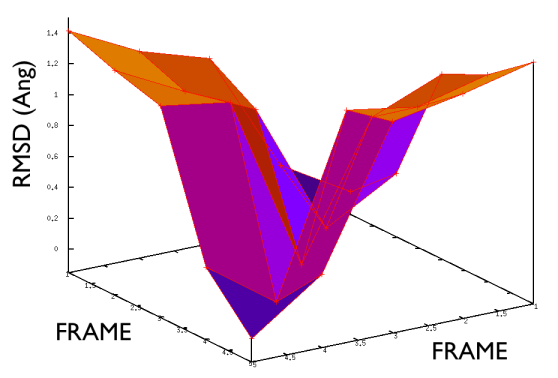A bad matrix for path variables is rather irregular and shows that some non neighbor frames are closer than the neighbor frames.

Aside from the general shape, which is important to keep the conformation-to-index relation (this, as we will see in the next part is crucial in the multidimensional scaling) the crucial thing is the distance between neighbors.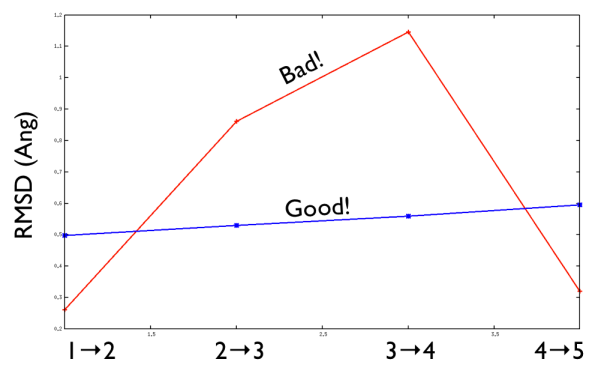Comparison of neighbor distance: the bad case has a nearest neighbor fluctuation of 0.8 units which is much more than the good case where the distance is much more uniform

As a matter of fact, this is not much important in the analysis but is truly crucial in the bias. When biasing a simulation, you generally want to introduce a force that push your system somewhere. In particular, when you add a bias which is based on a path collective variable, most likely you want that your system goes back and forth along your path. The bias is generally applied by an additional term in the Hamiltonian, this can be a spring term for Umbrella Sampling, a Gaussian function for Metadynamics or whatever term which is a function of the collective variable $$s$$. Therefore the Hamiltonian $$H (X)$$ where $$X$$ is the point of in the phase space where your system is takes the following form

$H'(X)=H(X)+U(S(X))$

where $$U(S(X))$$ is the force term which depends on the collective variable that ultimately is a function of the $$X$$. Now, when you use biased dynamics you need to evolve according the forces that this term produces (this only holds for MD, while not in MC) and therefore you need

$F_i=-\frac{d H'(X) }{d x_i} = -\frac{d H'(X) }{d x_i} -\frac{\partial U(S(X)) }{ \partial S}\frac{\partial S(X)}{\partial x_i}$

This underlines the fact that, whenever $$\frac{\partial S(X)}{\partial x_i}$$ is zero, then you have no force on the system. Now the derivative of the progress along the path is

$\frac{\partial S(X) }{\partial x_i} =\frac{\sum_{i=1}^{N} -\lambda\ i\ \frac{\partial \vert X-X_i \vert}{ \partial x_i} \exp^{-\lambda \vert X-X_i \vert }}{ \sum_{i=1}^{N} \exp^{-\lambda \vert X-X_i \vert } } - \frac{ (\sum_{i=1}^{N} i\ \exp^{-\lambda \vert X-X_i \vert } ) (\sum_{j=1}^{N} -\lambda \frac{\partial \vert X-X_j \vert}{ \partial x_i} \exp^{-\lambda \vert X-X_j \vert } ) }{ ( \sum_{i=1}^{N} \exp^{-\lambda \vert X-X_i \vert } )^2} = \frac{\sum_{i=1}^{N} -\lambda\ i\ \frac{\partial \vert X-X_i \vert}{ \partial x_i} \exp^{-\lambda \vert X-X_i \vert }}{ \sum_{i=1}^{N} \exp^{-\lambda \vert X-X_i \vert } } -s(X) \frac{ (\sum_{j=1}^{N} -\lambda \frac{\partial \vert X-X_j \vert}{ \partial x_i} \exp^{-\lambda \vert X-X_j \vert } ) } {\sum_{i=1}^{N} \exp^{-\lambda \vert X-X_i \vert } }$

which can be rewritten as

$\frac{\partial S(X) }{\partial x_i} = \lambda \frac{\sum_{i=1}^{N} \frac{\partial \vert X-X_i \vert}{ \partial x_i} \exp^{-\lambda \vert X-X_i \vert } [ s(X) - i ] } { \sum_{i=1}^{N} \exp^{-\lambda \vert X-X_i \vert } }$

It is interesting to note that whenever the $$\lambda$$ is too small the force will vanish. Additionally, when $$\lambda$$ is too large, then it one single exponential term will dominate over the other on a large part of phase space while the other will vanish. This means that the $$S(X)$$ will assume almost discrete values that produce zero force. Funny, isn't it?

# How many frames do I need?

A very common question that comes is the following: "I have my reaction or a model of it. how many frames do I need to properly define a path collective variable?" This is a very important point that requires a bit of thinking. It all depends on the limiting scale in your reaction. For example, if in your process you have a torsion, as the smallest event that you want to capture with path collective variable, then it is important that you mimic that torsion in the path and that this does not contain simply the initial and final point but also some intermediate. Similarly, if you have a concerted bond breaking, it might be that all takes place in the range of an Angstrom or so. In this case you should have intermediate frames that cover the sub-Angstrom scale. If you have both in the same path, then the smallest dominates and you have to mimic also the torsion with sub-Angstrom accuracy.

# Some tricks of the trade: the neighbors list.

If it happens that you have a very complex and detailed path to use, say that it contains 100 frames with 200 atoms each, then the calculation of a 100 alignment is required every time you need the CV. This can be quite expensive but you can use a trick. If your trajectory is continuous and you are sure that your trajectory does not show jumps where your system suddenly move from the reactant to the product, then you can use a so-called neighbor list. The plumed input shown before then becomes

p1: PATHMSD REFERENCE=all.pdb LAMBDA=50.0 NEIGH_STRIDE=100 NEIGH_SIZE=10
PRINT ARG=p1.sss,p1.zzz STRIDE=100 FILE=colvar FMT=%8.4f


and in this case only the closest 10 frames from the path will be used for the CV. Then the list of the frames to use is updated every 100 steps. If you are using a biased dynamics this may introduce sudden change in the derivatives, therefore it is better to check the stability of the setup before running production-quality calculations.

# The molecule of the day: alanine dipeptide

Here and probably in other parts of the tutorial a simple molecule is used as a test case. This is alanine dipeptide in vacuum. This rather simple molecule is useful to make many benchmark that are around for data analysis and free energy methods. It is a rather nice example since it presents two states separated by a high (free) energy barrier.

In Fig. belfast-2-ala-fig its structure is shown.The molecule of the day: alanine dipeptide.

The two main metastable states are called $$C_7eq$$ and $$C_7ax$$.Two metastable states of alanine dipeptide are characterized by their Ramachandran dihedral angles.

Here metastable states are intended as states which have a relatively low free energy compared to adjacent conformation. At this stage it is not really useful to know what is the free energy, just think in term of internal energy. This is almost the same for such a small system which so few degrees of freedom.

It is conventional use to show the two states in terms of Ramachandran dihedral angles, which are denoted with $$\Phi$$ and $$\Psi$$ in Fig. belfast-2-transition-fig .The Free energy landscape of alanine dipeptide in Ramachandran angles in the CHARMM27 force field.

# Examples

Now as a simple example, I want to show you that plotting some free dynamics trajectories shoot from the saddle point, you get a different plot in the path collective variables if you use the right path or if you use the wrong path.

In Fig. belfast-2-good-bad-path-fig I show you two example of possible path that join the $$C_7eq$$ and $$C_7ax$$ metastable states in alanine dipeptide. You might clearly expect that real (rare) trajectories that move from one basin to the other would rather move along the black line than on the red line.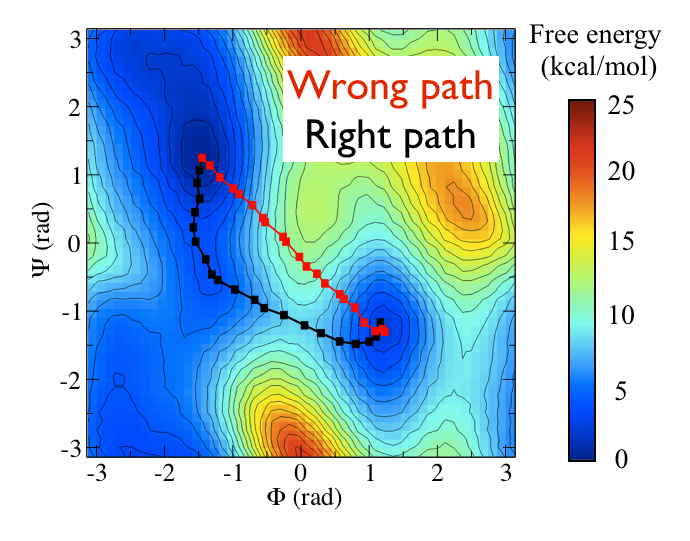Example of good and bad path: one sits confortably in the minimum free energy path connecting the two metastable states, while the other makes a direct connection via high energy states

So, in this example we do a sort of "commmittor analysis" where we start shooting a number of free molecular dynamics from the saddle point located at $$\Phi=0$$ and $$\Psi=-1$$ and we want to see which way do they go. Intuitively, by assigning random velocities every time we should find part of the trajectories that move toward $$C_7eq$$ and part that move towards $$C_7ax$$.

I provided you with two directories, each containing a bash script script.sh whose core (it is a bit more complicated in practice...) consists in:

#
# set how many runs you want to do
#
ntests=50
for i in seq 1 $ntests do # # assign a random velocity at each timestep by initializing the # sed s/SEED/$RANDOM/ md.mdp >newmd.mdp
#
# do the topology: this should write a topol.tpr
#
$GROMPP -c start.gro -p topol.top -f newmd.mdp$GROMACS_BIN/$MDRUN -plumed plumed.dat mv colvar colvar_$i
done


This runs 50 short MD runs (few hundreds steps) and just saves the colvar file into a labeled colvar file. In each mdrun plumed is used to plot the collective variables and it is something that reads like the following:

# Phi
t1: TORSION ATOMS=5,7,9,15
# Psi
t2: TORSION ATOMS=7,9,15,17
# The right path
p1: PATHMSD REFERENCE=right_path.dat LAMBDA=15100.
# The wrong path
p2: PATHMSD REFERENCE=wrong_path.dat  LAMBDA=8244.4
# Just a printout of all the stuff calculated so far
PRINT ARG=* STRIDE=2 FILE=colvar FMT=%12.8f


where I just want to plot $$\Phi$$ , $$\Psi$$ and the two path collective variables. Note that each path has a different LAMBDA parameters. Here the Ramachandran angles are plotted so you can realize which path is the system walking in a more comfortable projection. This is of course fine in such a small system but whenever you have to deal with larger systems and control hundreds of CVs at the same time, I think that path collective variables produce a more intuitive description for what you want to do.

If you run the script simply with

pd@plumed:~> ./script.sh


then after a minute or so, you should have a directory which is full of colvar files. Let's revise together how the colvar file is formatted:

#! FIELDS time t1 t2 p1.sss p1.zzz p2.sss p2.zzz
#! SET min_t1 -pi
#! SET max_t1 pi
#! SET min_t2 -pi
#! SET max_t2 pi
0.000000  -0.17752998  -1.01329788  13.87216908   0.00005492  12.00532256   0.00233905
0.004000  -0.13370142  -1.10611136  13.87613508   0.00004823  12.03390658   0.00255806
0.008000  -0.15633049  -1.14298481  13.88290617   0.00004511  12.07203319   0.00273764
0.012000  -0.23856451  -1.12343958  13.89969608   0.00004267  12.12872544   0.00284883
...


In first column you have the time, then t1 ( $$\Phi$$) , then t2 ( $$\Psi$$ ) and the path collective variables p1 and p2. Note that the action PATHMSD calculates both the progress along the path (p1.sss) and the distance from it (p1.zzz) . In PLUMED jargon, these are called "components". So a single Action (a line in plumed input) can calculate many components at the same time. This is not always the case: sometimes by default you have one component but specific flags may enable more components to be calculated (see DISTANCE for example). Note that the header (all the part of a colvar file that contains # as first character) is telling already what it inside the file and eventually also tells you if a variable is contained in boundaries (for example torsion angles, are periodic and their co-domain is defined in -Pi and Pi ).

At the end of the script, you also have two additional scripts. One is named script_rama.gplt and the other is named script_path.gplt. They contain some gnuplot commands that are very handy to visualize all the colvar files without making you load one by one, that would be a pain.

Now, let's visualize the result from the wrong path directory. In order to do so, after having run the calculation, then do

pd@plumed:~>gnuplot


what you see is that all the trajectories join the reactant and the product state along the low free energy path depicted before.

Now if you try to load the same bunch of trajectories as a function of the progress along the path and the distance from the path in the case of the wrong path then simply do

gnuplot> load "script_path_wrong.gplt"


What do you see? A number of trajectories move from the middle towards the right bottom side at low distance from the path. In the middle of the progress along the path, you have higher distance. This is expected since the distance zero corresponds to a unlikely, highly-energetic path which is unlikely to occur. Differently, if you now do

gnuplot> load "script_path_right.gplt"


You see that the path, compared to what happened before, run much closer to small distance from the path. This means that the provided path is right and more representative of what happens in the reactive path.

Note that going at high distances can be also beneficial. It might help you to explore alternative paths that you have not explored before. But beware, the more you get far from the path, the more states are accessible, in a similar way as the fact that the surface of a sphere increase with its radius. The surface ramps up much faster than the radius therefore you have a lots of states there. This means also high entropy, so many systems actually tend to drift to high distances while, on the contrary, zero distance is never reached in practice (zero entropy system is impossible to reach at finite temperature). So you can see by yourself that this can be a big can of worms. In particular, my experience with path collective variables and biological systems tells me that most of time is hopeless to go to high distances to find new path in many cases (for example, in folding). While this is worth whenever you think that the paths are not too many (alternative routes in chemical reaction or enzymatic catalysis).

# How to format my input?

Very often it is asked how to format a set of pdb to be suitably used with path collective variables. Here are some tricks.

• When you dump the files with vmd or (for gromacs users, using trjcat), the pdb you obtain is re-indexed from 1. This is also the case when you select a sub-ensemble of atoms of the path (e.g. the heavy atoms only or the backbone atoms). This is rather unfortunate and you have to fix is someway. My preference is to dump the whole pdb but water (when I do not need it) and use some awk script to select the atoms I am interested in.
• Pay attention to the last two column. These are occupancy and beta. With the first (occupancy) you set the atoms which are used to perform the alignment. The atoms which have zero occupancy will not be used in the alignment. The second column is beta and controls which atoms are used for the calculation of the distance after having performed the alignment on the set of atoms which have nonzero occupancy column. In this way you can align all your system by using a part of the system and calculate the distance respect to another set. This is handy in case of protein-ligand. You set the alignment of the protein and you calculate the distance based on the ligand and the part of the protein which is in contact with the protein. This is done for example in this article.
• Plumed-GUI (version > 2.0) provides the Structure->Build reference structure... function to generate inputs that conform to the above rules from within VMD.
• Note that all the atoms contained in the REFERENCE must be the same. You cannot have a variable number of atoms in each pdb contained in the reference.
• The reference is composed as a set of concatenated PDB files that are interrupted by a TER/END/ENDMDL card. Both HETATM and ATOM cards denote the atoms of the set.
• Include in the reference frames only the needed atoms. For example, if you have a methyl group involved in a conformational transition, it might be that you do not want to include the hydrogen atoms of the methyl since these rotate fast and probably they do not play ant relevant role.

# Fast forward: metadynamics on the path

This section is actually set a bit forward but I included here for completeness now. It is recommended to be read after you have an introduction on Metadynamics and to well-tempered Metadynamics in particular. Here I want to show a couple of concept together.

• Path collective variables can be used for exploring alternative routes. It is effective in highly structure molecules, while it is tricky on complex molecules whenever you have many competing routes
• Path collective variables suffer from problems at the endpoints (as the highly popular coordinates COORDINATION for example) that can be cured with flexible hills and an appropriate reweighting procedure within the well-tempered Metadynamics scheme.

Let's go to the last problem. All comes from the derivative belfast-2-sder-eq. Whenever you have a point of phase space which is similar to one of the endpoint than one of the points in the center then you get a $$s(X)$$ which is 1 or N (where N is the number of frames composing the path collective variable). In this case that exponential will dominate the others and you are left with a constant (since the derivative of RMSD is a constant since it is linear in space). This means that, no matter what happens here, you have small force. Additionally you have small motion in the CV space. You can move a lot in configuration space but if the closest point is one of the endpoint, your CV value will always be one of the endpoint itself. So, if you use a fixed width of your CV which you retrieve from a middle point in your path, this is not suitable at all at the endpoints where your CV fluctuates much less. On the contrary if you pick the hills width by making a free dynamics on the end states you might pick some sigmas that are smaller than what you might use in the middle of the path. This might give a rough free energy profile and definitely more time to converge. A possible solution is to use the adaptive gaussian width scheme. In this scheme you adapt the hills to their fluctuation in time. You find more details in  Additionally you also adopt a non spherical shape taking into account variable correlation. So in this scheme you do not have to fix one sigma per variable sigma, but just the time in which you calculate this correlation (another possibility is to calculate it from the compression of phase space but will not be covered here). The input of metadynamics might become something like this

t1: TORSION ATOMS=5,7,9,15
t2: TORSION ATOMS=7,9,15,17
p1: PATHMSD REFERENCE=right_path.dat LAMBDA=15100.
p2: PATHMSD REFERENCE=wrong_path.dat  LAMBDA=8244.4
#
# do a metadynamics on the right path, use adaptive hills whose decay time is 125 steps (250 fs)
# and rather standard WT parameters
#
PRINT ARG=* STRIDE=10 FILE=colvar FMT=%12.8f


You can find this example in the directory BIASED_DYNAMICS. After you run for a while it is interesting to have a feeling for the change in shape of the hills. That you can do with

pd@plumed:~> gnuplot
gnuplot>  p "<head -400 HILLS" u 2:3:4:5 w xyer


that plots the hills in the progress along the path and the distance from the path and add an error bar which reflects the diagonal width of the flexible hills for the first 400 hills (Hey note the funny trick in gnuplot in which you can manipulate the data like in a bash script directly in gnuplot. That's very handy!).The hills width as error bar as function of progress and distance from the path.

There are a number of things to observe: first that the path explores high distance since the metadynamics is working also in the distance from the path thus accessing the paths that were not explored before, namely the one that goes from the upper left corner of the Ramachandran plot and the one that passes through the lower left corner. So in this way one can also explore other paths. Additionally you can see that the hills are changing size rather considerably. This helps the system to travel faster since at each time you use something that has a nonzero gradient and your forces act on your system in an effective way. Another point is that you can see broad hills covering places which you have not visited in practice. For example you see that hills extend so wide to cover point that have negative progress along the path, which is impossible by using the present definition of the progress along the path. This introduced a problem in calculating the free energy. You actually have to correct for the point that you visited in reality.

You can actually use sum_hills to this purpose in a two-step procedure. First you calculate the negative bias on a given range:

pd@plumed:~> plumed sum_hills --hills HILLS --negbias  --outfile negative_bias.dat --bin 100,100 --min -5,-0.005 --max 25,0.05


and then calculate the correction. You can use the same hills file for that purpose. The initial transient time should not matter if your simulation is long enough to see many recrossing and, secondly, you should check that the hills height in the well tempered are small compared to the beginning.

pd@plumed:~> plumed sum_hills --histo HILLS --bin 100,100 --min -5,-0.005 --max 25,0.05 --kt 2.5 --sigma 0.5,0.001 --outhisto correction.dat


Note that in the correction you should assign a sigma, that is a "trust radius" in which you think that whenever you have a point somewhere, there in the neighborhood you assign a bin (it is done with Gaussian in reality, but this does not matter much). This is the important point that compensates for the issues you might encounter putting excessive large hills in places that you have not visited. It is nice to have a look to the correction and compare with the hills in the same range.

gnuplot> set pm3d
gnuplot> spl "correction.dat" u 1:2:3 w l
gnuplot> set contour
gnuplot> set cntrp lev incremental -20,4.,1000.
gnuplot> set view map
gnuplot> unset clabel
gnuplot> replot


You might notice that there are no contour in the unrealistic range, this means that the free energy correction is impossible to calculate since it is too high (see Fig. belfast-2-metadpath-correction-fig ).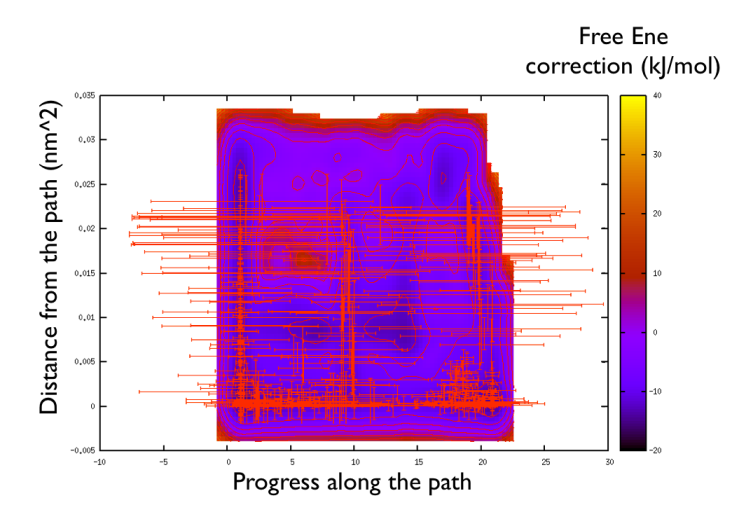Comparison of the free energy correction with the first 400 deposited hills. The excessive range has too high energy and is cut out from the correction. Isolines are every 4 kJ/mol.

Now the last thing that one has to do to have the most plausible free energy landscape is to sum both the correction and the negative bias produced. This can be easily done in gnuplot as follows:

gnuplot> set pm3d
gnuplot> spl "<paste negative_bias.dat correction.dat " u 1:2:($3+$8) w pm3d
gnuplot> set view map
gnuplot> unset key
gnuplot> set xr [-2:23]
gnuplot> set contour
gnuplot> unset clabel
gnuplot> set cbrange [-140:-30]
gnuplot> set cntrp lev incr -140,6,-30Final free energy obtained by the sum of the negative bias and the correction. Isolines are every 6 kJ/mol.

So now we can comment a bit on the free energy surface obtained and note that there is a free energy path that connects the two endpoints and runs very close to zero distance from the path. This means that our input path is resembles what is really happening in the system. Additionally you can see that there are many comparable routes different from the straight path. Can you make a sense of it just by looking at the free energy on the Ramachandran plot?